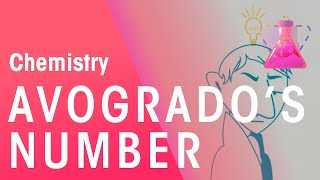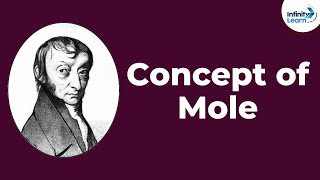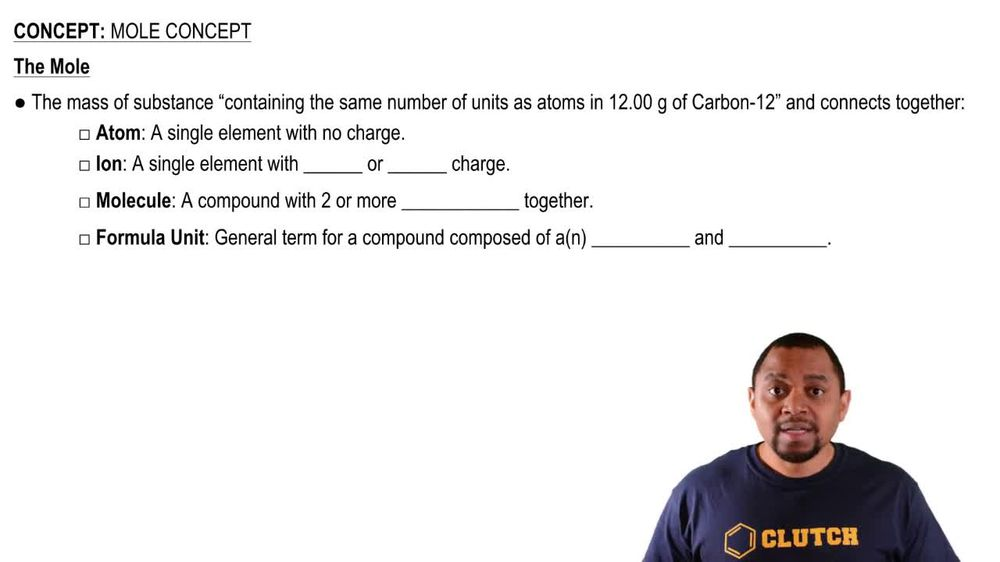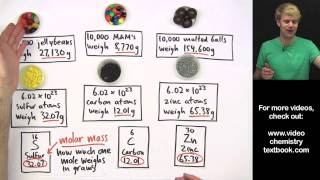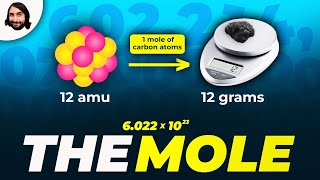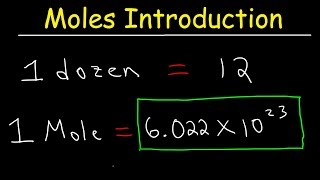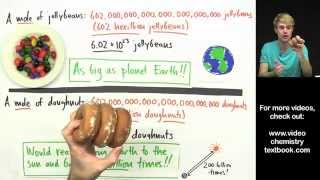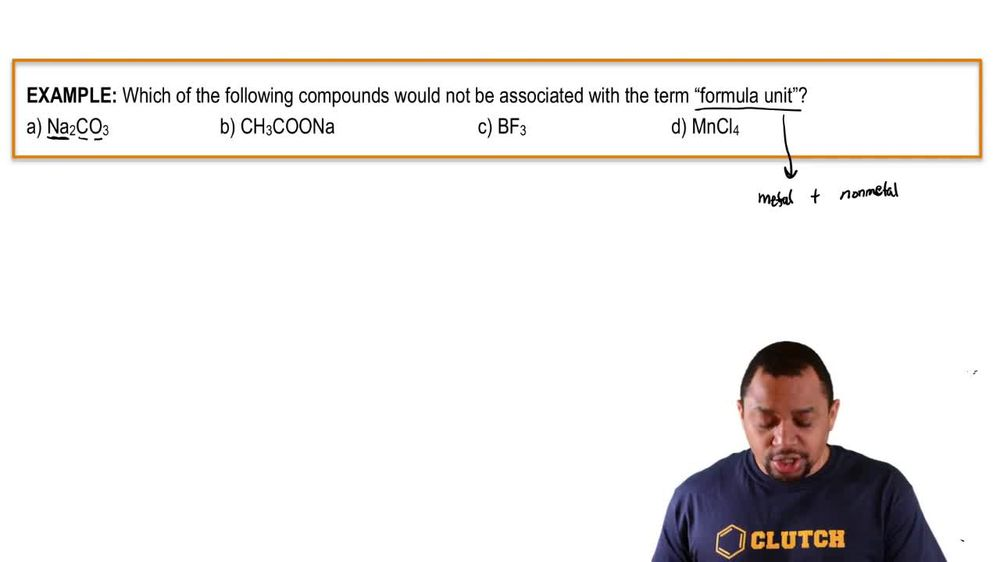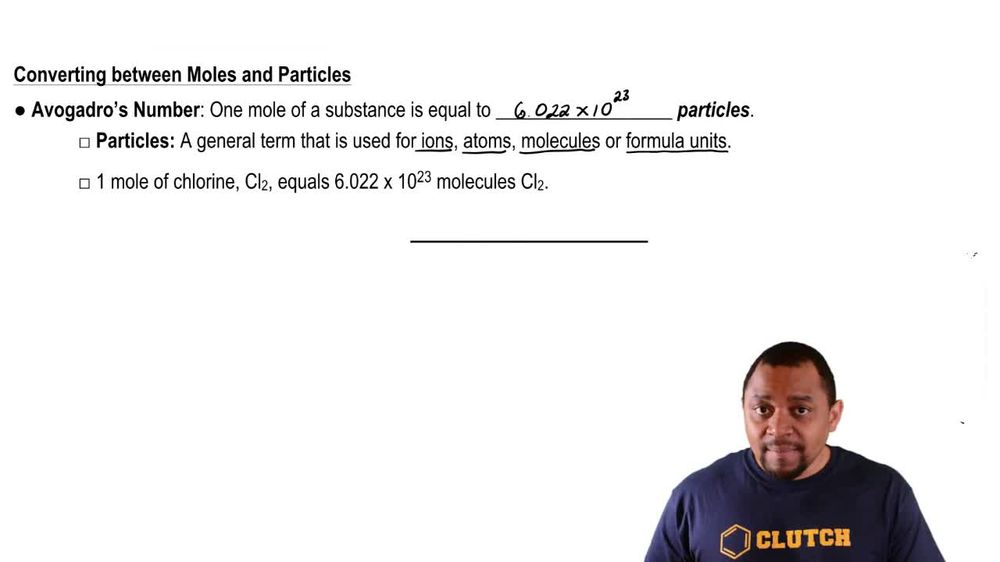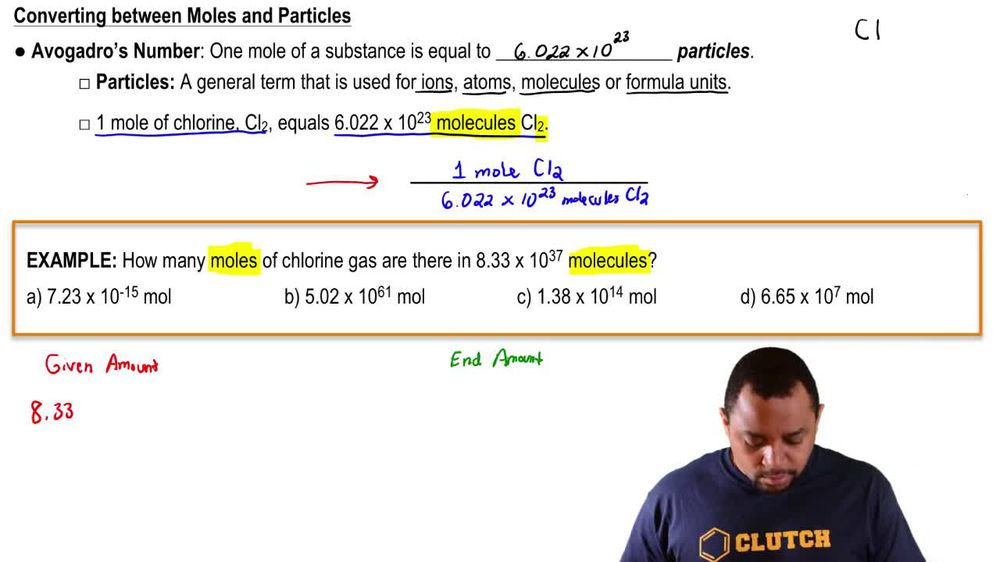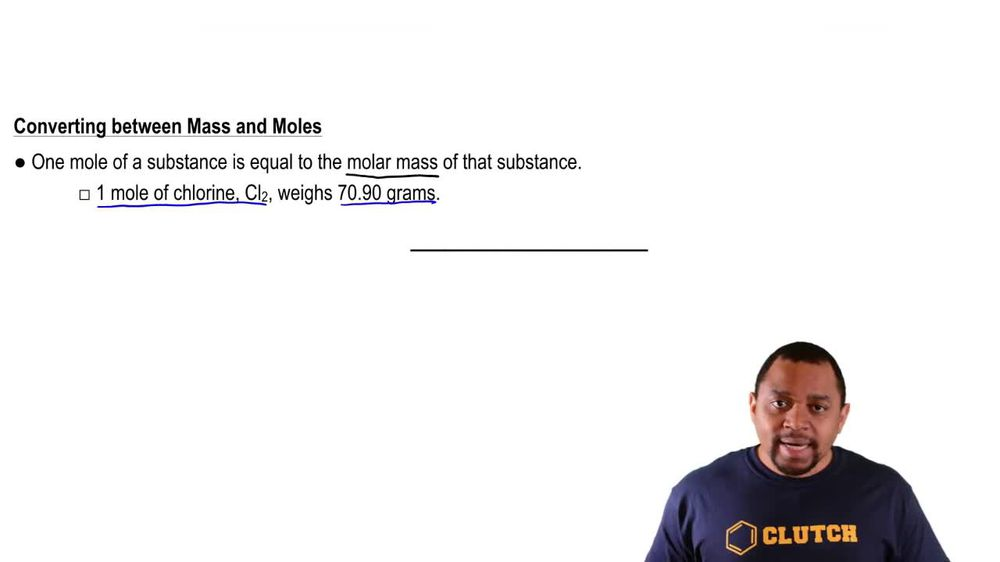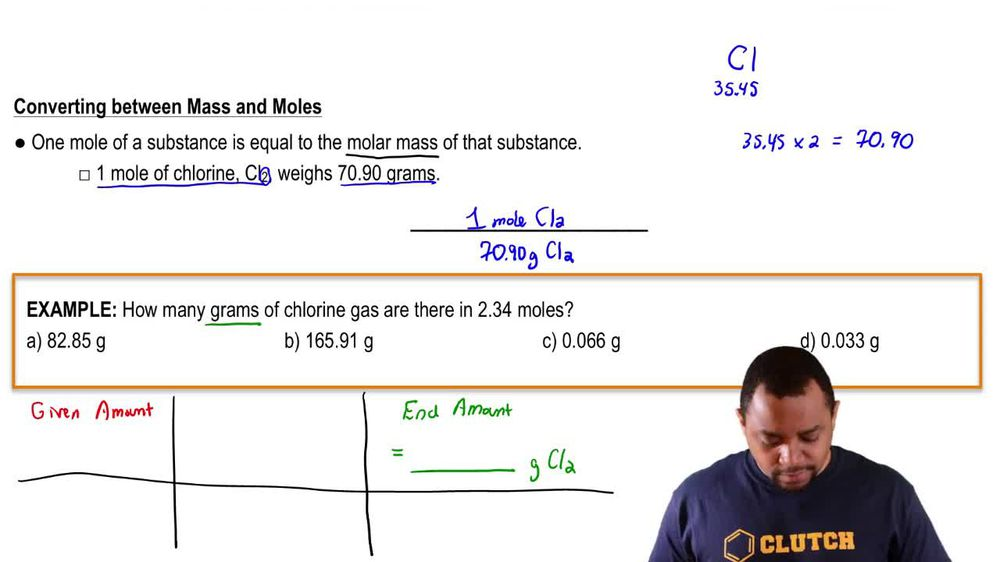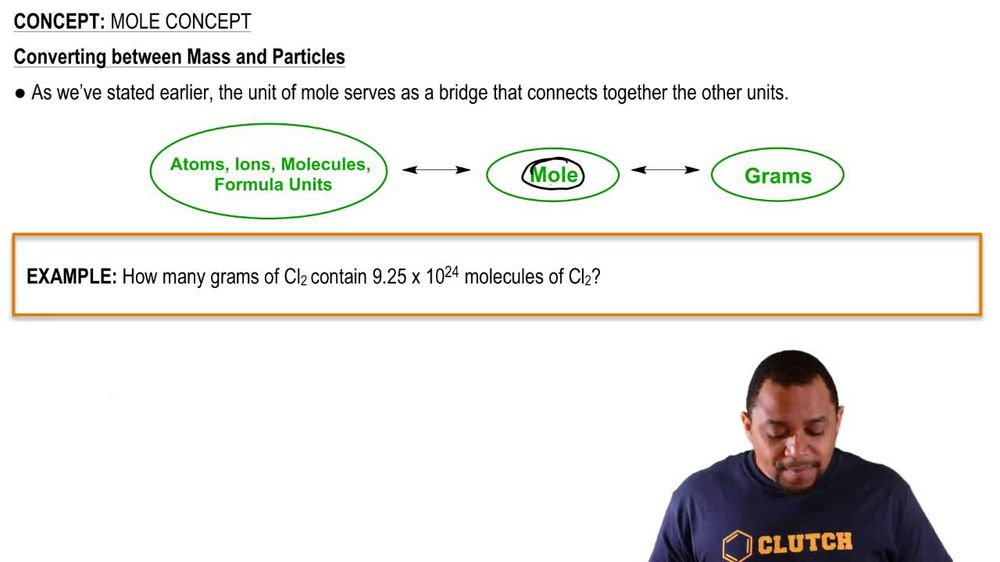Start typing, then use the up and down arrows to select an option from the list.
1. 2. Atoms & Elements2. Mole Concept
Problem

# A sample of glucose, C6H12O6, contains 1.250 * 1021 carbon atoms. (c) How many moles of glucose does it contain?

Relevant Solution1m
Play a video:
Hey everyone, we're asked to consider a sample of nicotine that was observed to have 3.561 times 10 to the 25 nitrogen atoms were asked to calculate the number of moles of nicotine in the sample for this question, let's first write out our pathway. We were given nitrogen atoms will then have to convert into mole of nitrogen by using avocados number. And next we can use our multiple ratios to get to mole of nicotine. Now that we've written out our pathway, let's go ahead and use our dimensional analysis to answer this question, starting with 3.561 times 10 to the nitrogen atoms. We're going to use avocados number here and we know that we have 6.22 times 10 to 23rd Atoms of nitrogen per one mole of nitrogen. Next, using our multiple ratios, we can see that we have two mole of nitrogen in one mole of our nicotine and we can see that through the subscript of two in our formula. So calculating this out and canceling out all of our units, We end up with an answer of 29. mole of nicotine. Now, I hope this made sense. And let us know if you have any questions